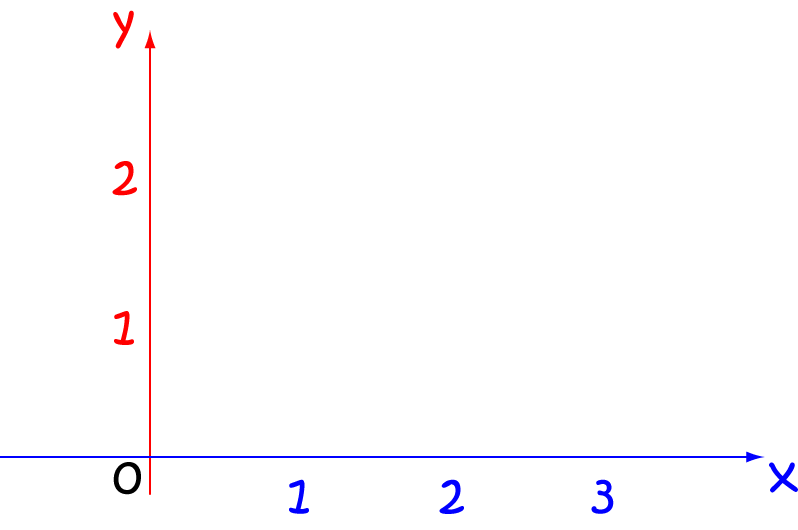# Definition of y-axisThe $xy$-plane has two lines called axes that pass through the origin (the point $(0,0)$) and help you to measure how far to the left or right and how far up or down a point lies in the plane.

The $y$-axis is the vertical line in the plane that passes through the origin (the point $(0,0)$) and helps you to measure how far up or down the plane a point lies.

### Description

The aim of this dictionary is to provide definitions to common mathematical terms. Students learn a new math skill every week at school, sometimes just before they start a new skill, if they want to look at what a specific term means, this is where this dictionary will become handy and a go-to guide for a student.

### Audience

Year 1 to Year 12 students

### Learning Objectives

Learn common math terms starting with letter Y

Author: Subject Coach
You must be logged in as Student to ask a Question.